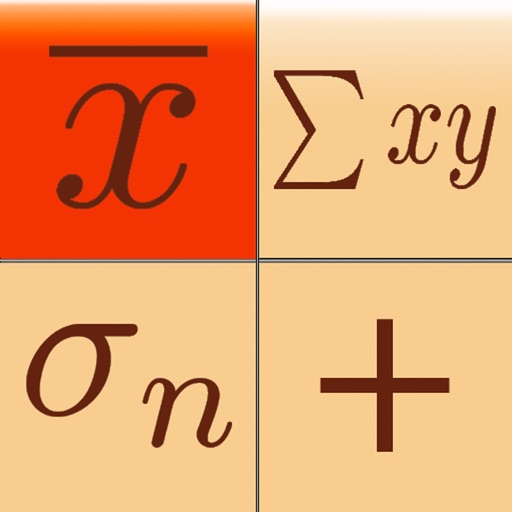## Statistics Calculator-- is an introductory app for our flagship Statistics Calculator++, a calculator with great advanced scientific features as well as statistical, regression analysis, probability distributions, confidence intervals, and hypothesis tests capabilities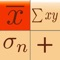# Statistics Calculator--

by Dogahe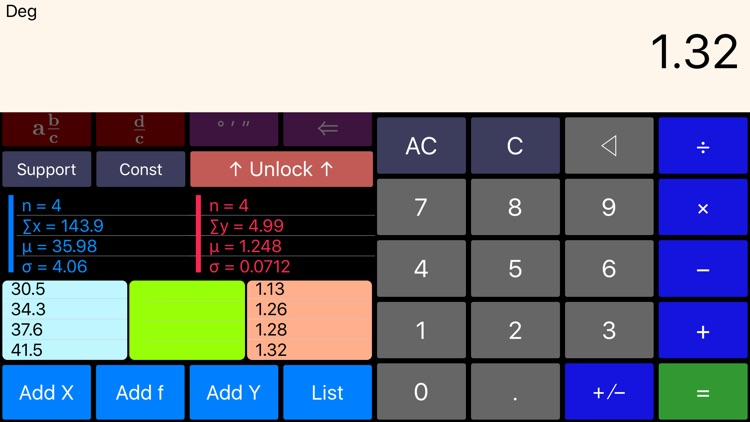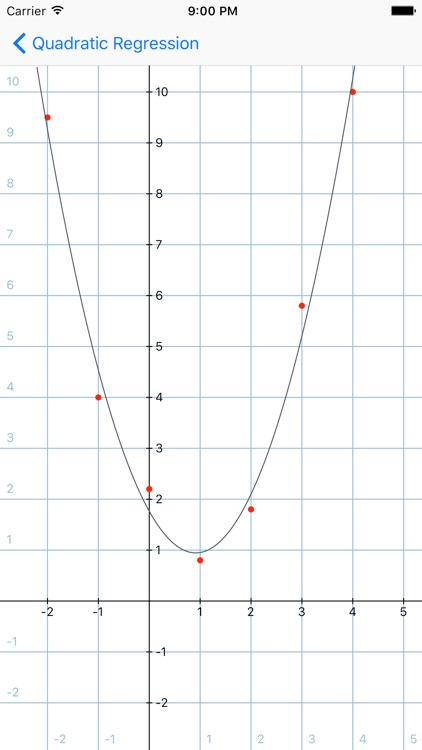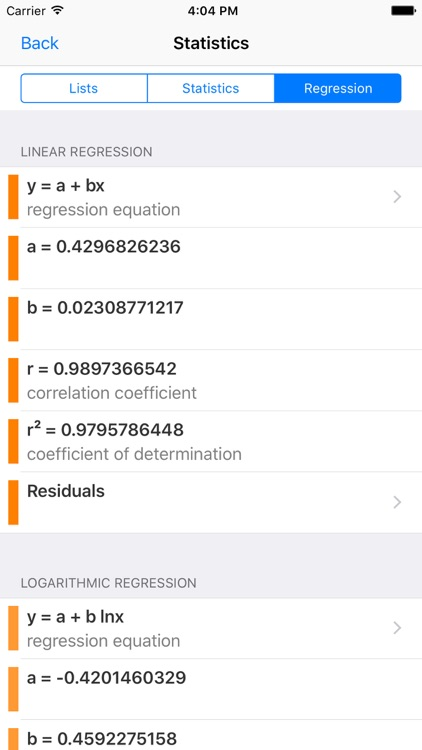Statistics Calculator-- is an introductory app for our flagship Statistics Calculator++, a calculator with great advanced scientific features as well as statistical, regression analysis, probability distributions, confidence intervals, and hypothesis tests capabilities.### App Details

Version
1.03
Rating
(149)
Size
12Mb
Genre
Utilities Education
Last updated
September 13, 2020
Release date
December 13, 2016

### Statistics Calculator is FREE but there are more add-ons

• \$0.99

Scientific Plus

• \$0.99

List Size Plus

• \$0.99

Graphing Regression Models

• \$0.99

### App Screenshots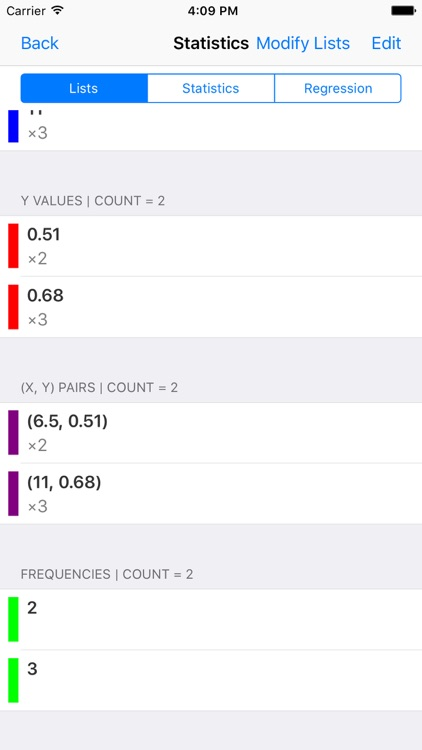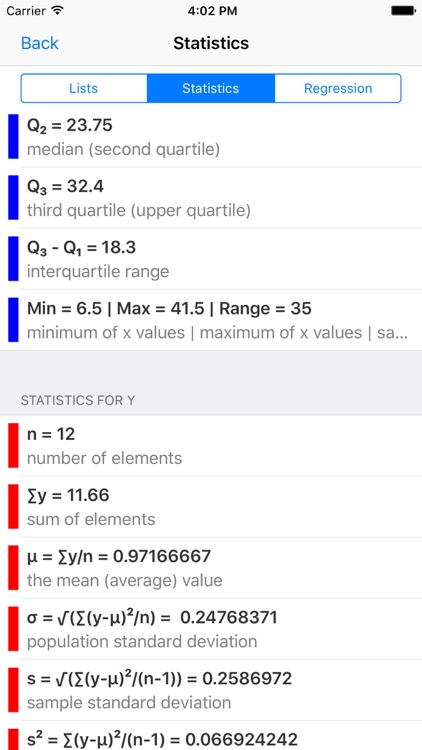### App Store Description

Statistics Calculator-- is an introductory app for our flagship Statistics Calculator++, a calculator with great advanced scientific features as well as statistical, regression analysis, probability distributions, confidence intervals, and hypothesis tests capabilities.

Statistics Calculator-- will have all the functionality of Statistics Calculator++ by purchasing a single In-App Purchase.

The purchase will remove the limit on the size of the lists, unlocks the advanced scientific features, graphs the regression models, and unlocks probability distributions, confidence intervals, and hypothesis tests capabilities.

In designing the statistics functions of this app we had one thing in mind: The user should not need to read a single line of manual to perform the statistical analysis.

Simply use Add X, Add Y, and Add f buttons to enter the elements of the lists and frequencies, if any. And you are done. All the statistics and regression models are only a few taps and swipes away. Tap the List button to see the lists entered. The elements of the lists are interpreted independently (as two separate lists) and jointly for regression analysis (if the size of the lists are equal).

The sum, mean, population standard deviation, sample standard deviation, sample variance, sum of squares, min, max, quartiles, and sum of multiplications of two lists are presented in a table. Each statistics measure can be chosen from the table and used for further calculations.

For regression analysis linear regression, logarithmic regression, exponential regression, power regression, and quadratic regression (through an additional In-App Purchase) are calculated. Statistics Calculator-- has an extremely simple and intuitive user interface to choose the regression model and calculate predictions for x and y values and view residuals and residual sum of squares.

Other major features of Statistics Calculator-- are:

- The left pane scrolls to reveal more functionality without a need to switch modes. The digits and most common operations are always visible and the less common operations can be revealed by scrolling the left half of the screen.
- Shows the expression as you enter and keeps a history of your calculations (Swipe up on the screen to reveal the history)
- Backspace to delete last digit entered.
- Sinusoidal functions can be calculated for angles in units of degrees, radians, or gradians.

The following features are available through Advanced Scientific In-App Purchase.
- Capability of performing fraction manipulations and converting between different formats (proper, improper, and mixed fractions) and seamless integration of fraction, integer, and decimal manipulations.
- Capability of inputting degrees, minutes, and seconds for angles and converting between degrees-minutes-seconds format and decimal format.
- Capability of calculating permutation, combination, Least Common Multiple (LCM), Greatest Common Divisor (GCD), and prime factorization.
- Quadratic and system of two linear equations solver
- Rectangular to polar and polar to rectangular coordinate conversion (extremely useful for phasor calculations for electrical engineers)
- Throws a set of dice (choice of 1 die to 4 dice)

You are going to love the simplicity of the user interface and yet have the powerful functionality with which Statistics Calculator-- is loaded.